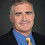## Monday, July 31, 2006

### Full Derandomization

What does the complexity world look like if we have hard functions that let us efficiently derandomize? All of the results in this post follow from the following open but reasonable hardness assumption.
There is some language L computable in time 2O(n) such that for some ε>0, every algorithm A computing L uses 2εn space for sufficiently large n.
First are the complexity class collapses. As always see the Zoo if some class does not look familiar.
• ZPP = RP = BPP = P
• MA = AM = NP. In particular Graph Isomorphism and all of statistical zero-knowledge lie in NP∩co-NP.
• S2P = ZPPNP = BPPNP = PNP
• The polynomial-time hierarchy lies in SPP. As a corollary PH ⊆ ⊕P ∩ ModkP ∩ C=P ∩ PP ∩ AWPP
One can also derandomize Valiant-Vazirani. There is a polynomial time procedure that maps a CNF formula φ to a polynomial list of formula ψi such that
1. If φ is not satisfiable then all of the ψi are not satisfiable.
2. If φ is satisfiable then some ψi has exactly one satisfying assignment.
Beyond complexity classes we also get some randomized constructions such as creating Ramsey graphs. In polynomial in n time, we can generate a polynomial list of graphs on n vertices, most of which have no clique or independent set of size 2 log n.

This gives a short list containing many Ramsey graphs. We don't know how to verify a Ramsey graph efficiently so even though we have the short list we don't know how to create a single one. Contrast this to the best known deterministic construction that creates a graph with no clique or independent set of size 2(log n)o(1).

We also get essentially optimal extractors. Given an distribution D on strings of length n where every string has probability at most 2-k and O(log n) additional truly random coins we can output a distribution on length k strings very close to uniform. The truly random coins are used both for the seed of the pseudorandom generator to create the extractor and in applying the extractor itself.

One also gets polynomial-time computable universal traversal sequences, a path that hits every vertex on any undirected graph on n nodes. The generator will give a polynomial list of sequences but one can just concatenate those sequences. The hardness assumption above won't put the sequence in log space, though we do believe such log-space computable sequences exist. Reingold's proof that we can decide undirected connectivity in logarithm space does not produce a universal traversal sequence though it does give a related exploration sequence.

There are many more implications of full derandomization including other complexity class inclusions, combinatorial constructions and some applications for resource-bounded Kolmogorov complexity.

1.keep hoping!

2.Can you please give more details on the short list of mostly ramsey graphs. What is "short list" and what is "most"?

3.By "short" I mean a polynomial (in the size of the graph) long list and by "most" I mean at least one will be Ramsey. A more careful analysis and/or allowing slightly larger independent sets and cliques and "most" would really mean "nearly all".

4.Where can I find a proof that that assumption indeed implies BPP = P? It is probably a classical paper (or set of papers) but I am not that familiar with complexity theory.

5.I like this survey by Peter Bro Miltersen.

6.Can you please give a reference to the ramsey thing you mentioned

7.8.One can also derandomize Polynomial Identity Testing, which is interesting since the work of Kabanets and Impagliazzo. Derandomization of this implies separating NEXP from P/poly.

Also it is not just all of statistical zero-knowledge lie in NP?co-NP, but also languages having interactive proof of log(n) statistical knowledge complexity will be in NP?co-NP. Languages having interactive proof in which prover sends bounded number of bits will also be in NP?co-NP?

9.What would be the applications for resource-bounded Kolmogorov complexity theory? Thanks!

10.Most notably you can derandomize Sipser's constructions to show that for any set A a subset of strings of length n, for all x in A, KD^poly(x|A) is at most log |A| + log log |A| + O(1).